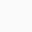# 1/(1+x^(b-a)+x^(c-a) )+1/(1+x^(a-b)+x^(c-b) )+1/(1+x^(b-c)+x^(a-c) )=1

The text form of this sum (1/ (1+x^(b-a)+x^(c-a) )+(1/(1+x^(a-b)+x^(c-b) )+(1/(1+x^(b-c)+x^(a-c) )=1 is given below. So that students may not get confused with the text form of the sum.

Watch video or see this solution:
First term: (1/ (1+x^(b-a)+x^(c-a) )
=(1/ (1+x^b*x^a)+x^c*x^a))
=(x^a/ (x^a+x^b+x^c))
Second term: (1/ (1+x^(a-b)+x^(c-b) )
=(1/ (1+x^a*x^b)+x^c*x^b))
=(x^b/ (x^a+x^b+x^c))
Third term: (1/ (1+x^(b-c)+x^(a-c) )
=(1/ (1+x^b*x^c)+x^a*x^c))
=(x^c/ (x^a+x^b+x^c))
Therefore
First term+Second term+third term
=(x^a/ (x^a+x^b+x^c))+(x^b/ (x^a+x^b+x^c))+(x^c/ (x^a+x^b+x^c))
=((x^a+x^b+x^c)/ (x^a+x^b+x^c))
=1
LHS=RHS
Proved
Its solution is also given in the video.
keywords:online mathematics, online mathematics degree, online mathematics courses, teaching mathematics.

##### 3 thoughts on “1/(1+x^(b-a)+x^(c-a) )+1/(1+x^(a-b)+x^(c-b) )+1/(1+x^(b-c)+x^(a-c) )=1”
1.Azra Ahmad says:

(2^(1 / 3)) * (3^(1 / 4)) =
1.65814935214
This is the direct value.
============
I think your some is incomplete.

2.Anonymous says:

simplify (2)^(1/3)*(3)^(1/4) Please solve this question

3.rahul karmakar says:

thanku